# Clustering[Spectral Clustering]

### 0. 背景

• 1 - 无向权重图：谱聚类是基于图论结构，也是数据结构的毗邻矩阵来实现的，即将所有的点的看成是一个相互连接的图（graph）；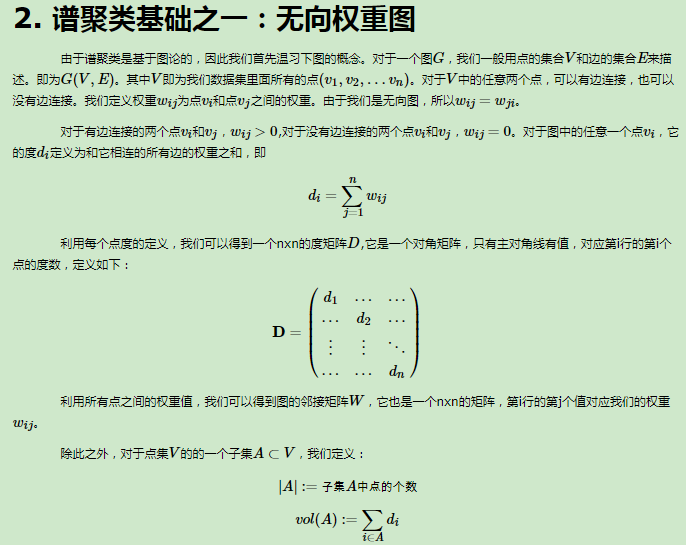上图中$|A|$即为聚类后的一个类别的数据点集合；
• 2 - 相似矩阵：通过定义相似函数，计算不同点之间的相似性，将其作为两点之间连线上的权重值。相距越近，则权重越大，越远则权重值越小；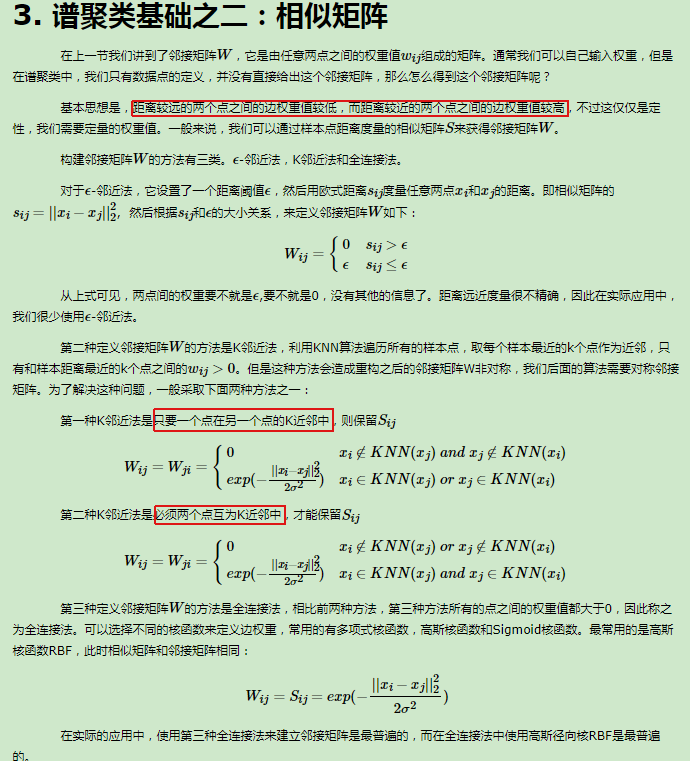其中第三种全连接方法的高斯方差决定了近邻的范围是多大；
• 3 - 最后当然就是想着怎么把图给切割开，fisher准则就是聚类的目的为：类内间距最小，类间间距最大。从而期望于切割整个图之后，子图之间连线的权重之和最小；

### 1. 谱聚类

#### 1.1 未归一化的图拉普拉斯矩阵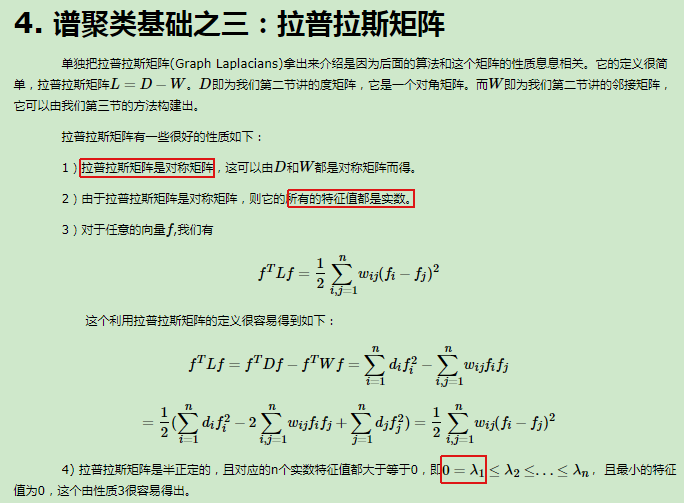• 一个实数对称矩阵的所有特征值都是实数(来自《introduction to linear algebra》chapter 6.4)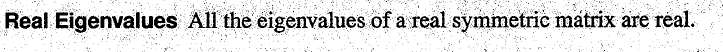• 证明一个矩阵是半正定的方式如下(来自《introduction to linear algebra》chapter 6.5)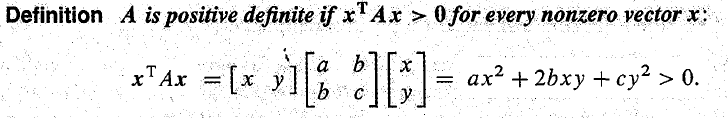• k=1:即整个图被归为1类，假设$f$是特征值0的特征向量，那么：因为$w_{ij}$是非负的，所以和为0的时候即$w_{ij}(f_i-f_j)^2$等于0，那么假设两个点是相连的，即$w_{ij}>0$，那么$f_i=f_j$。这样的话，可以发现对于所有可以通过某个路径进行相连的所有点，在$f$中的值都是相同的。那么如果只有一个类别，那么就只有一个向量。我们举个例子，假设有一个图，其中3个节点，两两相连，权重值都为1：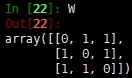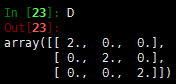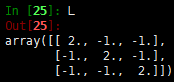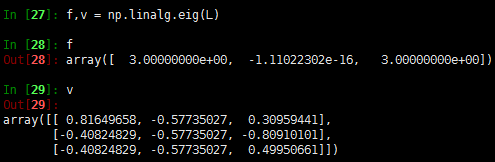可以看出，第二个特征值为0（近似），其对应的特征向量为$[-0.57,-0.57,-0.57]=-0.57*[1,1,1]$，而$[1,1,1]$被称为常量向量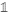• k=其他值。
不失一般性，假设图中的所有的点都是按照其对应的子图类进行有序排列的，在这种情况下，毗邻矩阵$W$可以变成一个块矩阵组成的形式，当然对于$L$也是一样的：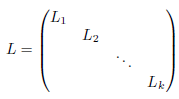可以发现每个块$L_i$都是一个对应的图拉普拉斯子矩阵，对应着第$i$个连通子图。那么依据矩阵的知识，相当于将整个矩阵划分成相同特性的多个子矩阵，其中$L$的特征向量就等于第$L_i$个特征向量的值加上其他子矩阵维度上为0。且$L_i$是一个联通图，那么其就有特征值为0且所有元素为1的特征向量。因此，矩阵$L$的子矩阵中特征值为0的个数就等于联通子图的个数。

#### 1.2 归一化的图拉普拉斯矩阵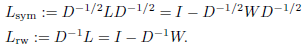$L_{sym}$$L_{rw}$的特性

• 1 - 对于任何向量$f\in R^n$，有：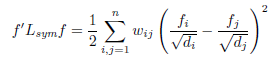• 2 - 有且仅有$\lambda$$w=D^{1/2}u$分别是$L_{sym}$的特征值和特征向量时，$\lambda$$u$就是是$L_{rw}$的特征值和特征向量；
• 3 - 有且仅有$\lambda$$u$满足$Lu=\lambda Du$时，$\lambda$$u$$L_{rw}$的特征值和特征向量
• 4 - 0和常量向量$L_{rw}$的特征值和特征向量，0和$D^{1/2}$$L_{sym}$的特征值和特征向量
• 5 - $L_{sym}$$L_{rw}$都是半正定的，且有n个非零的实值特征值，$0\leq \lambda_1 \leq \lambda_2 ...\leq \lambda_n$

• 对于$L_{rw}$，其0特征值对应的特征空间的基向量为这些子图对应的指示向量• 对于$L_{sym}$，其0特征值的特征空间的基向量为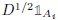### 2.聚类方法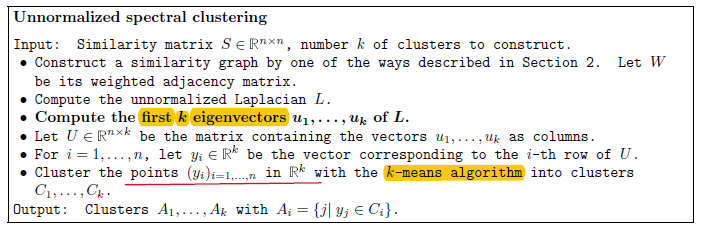• 1 - 选择合适的相似函数，计算相似矩阵
• 2 - 计算度矩阵$D$,毗邻权重矩阵$W$，从而建立拉普拉斯矩阵$L$
• 3 - 计算拉普拉斯矩阵$L$的前k个特征向量，组成矩阵$n\times k$
• 4 - 用k-mean算法将上述的特征向量矩阵按行为样本（即$n$个样本），列为特征(即k个特征)进行k个类别的聚类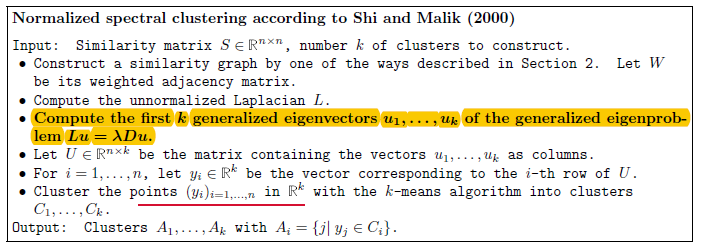• 上述图中，该算法使用的是$L$的广义特征向量。上述流程作用于$L_{rw}$，所以称为归一化的谱聚类。• 上述图中，该算法使用的是$L_{sym}$，并且添加了一个额外的行归一化,同样也是归一化的谱聚类。

【待续。。。】

[] 1 - Mohar, B. (1991). The Laplacian spectrum of graphs. In Graph theory, combinatorics, and applications. Vol. 2 (Kalamazoo, MI, 1988) (pp. 871 { 898). New York: Wiley
[] 2 - Mohar, B. (1997). Some applications of Laplace eigenvalues of graphs. In G. Hahn and G. Sabidussi (Eds.), Graph Symmetry: Algebraic Methods and Applications (Vol. NATO ASI Ser. C 497, pp. 225 { 275). Kluwer
[] 3 - Chung, F. (1997). Spectral graph theory (Vol. 92 of the CBMS Regional Conference Series in Mathematics). Conference Board of the Mathematical Sciences, Washington

posted @ 2018-03-16 09:42  仙守  阅读(1371)  评论(0编辑  收藏  举报